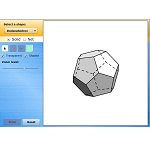A Non-profit Organization to Help Students Study, Explore, and Engage

Math 5, Alberta Curriculum

(Free Resources)

Shortcuts

General Practice on Nelson: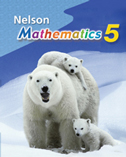Ideas for Teachers

Books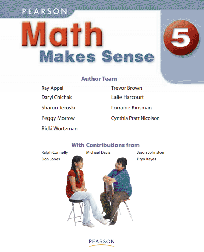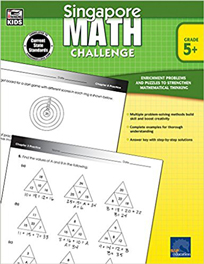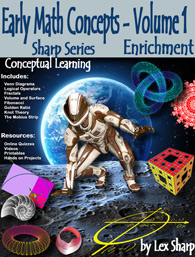See the Chapter on Fibonacci Sequence.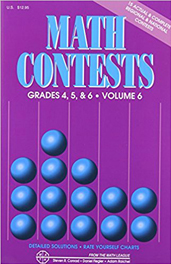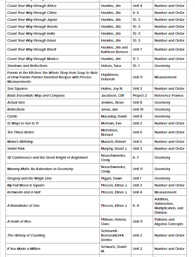University of Chicago, Grade 5 STEM Book List

Divisibility Rules

(Back to Shortcuts...)

Quick ways to determine divisibility:

A number is divisible by 2 if it terminates with 2, 4, 6, 8, or 0.
A number is divisible by 3 if the sum of its digits is divisible by 3.
A number is divisible by 4 if the last two digits are divisible by 4.
A number is divisible by 5 if it terminates with 5 or 0.
A number is divisible by 6 if it is divisible by both 2 and 3.
A number is divisible by 8 if the last three digits are divisible by 8.
A number is divisible by 9 if the sum of digits is divisible by 9.
A number is divisible by 10 if it terminates with 0.

Greatest Common Factor (GCF)

(Back to Shortcuts...)

Greatest Common Factor (GCF) Worksheets

http://www.mathatube.com/factors-worksheets.html
Factors of a Number worksheets   http://www.mathatube.com/factors-worksheets.html

Fractions

(Back to Shortcuts...)

Introduction to Fractions

The Intro on Khan Academy & Practice

on Fractions

Equivalent Fractions
Animation
http://www.learnalberta.ca/content/memg/division02/equivalent%20fractions/index.html

Patterns and Sequences

(Back to Shortcuts...)

Make patterns fun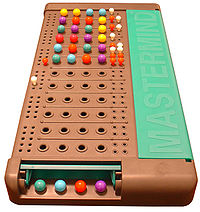Patterns - Lessons and Practice   https://courseware.cemc.uwaterloo.ca/27?gid=69

What's the general term of a sequence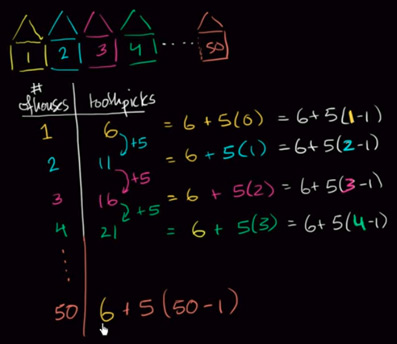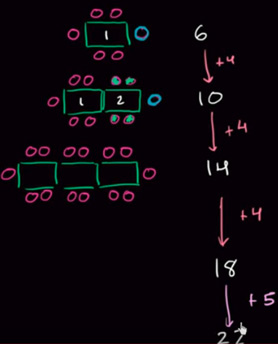More Practice...

Practice on Nelson: Patterns and Relationships   http://www.mathk8.nelson.com/math7/studentcentre/studtryout.html#ch4

Viual Patterns   http://www.visualpatterns.org/

Geometry

(Back to Shortcuts...)

Interactive Activity
Manipulate various geometric solids